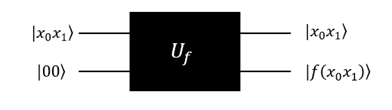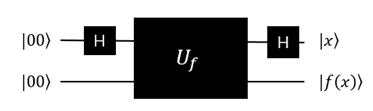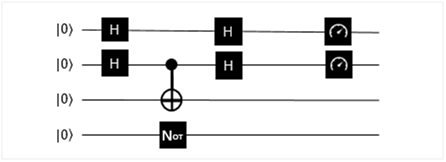6.6 Simon算法¶

6.6.1 Simon算法介绍¶

Simon问题是Daniel Simon在1994年提出。它是一个计算问题, 可以在量子计算机上以指数速度相较经典计算机更快地解决。虽然这个问题本身目前没有在实际应用中产生实际价值, 但它的趣味内涵在于它证明了量子算法可以比任何经典算法更快地解决这个问题。

Simon的算法也启发了Shor算法（详细请查阅Shor量子算法）。这两个问题都是阿贝尔隐子群（Abelian hidden subgroup problem）问题的特例, 而且是现今已知有效的量子算法。

问题描述：¶

• 给定一个方程：
$f:\{0,1\}^n→\{0,1\}^n$
• 存在 $$s∈\{0,1\}^n$$，对所有的 $$x,y∈\{0,1\}^n$$，满足下面的性质：
$$f(x)=f(y)$$ 当且仅当 $$x=y$$$$x⊕y=s$$

（这里⊕表示模2加。)

算法目标：¶

(1)s=01 (2)s=10 (3)s=11
x f(x) x f(x) x f(x)
00 1 00 1 00 1
01 1 01 2 01 3
10 0 10 1 10 3
11 0 11 2 11 1

量子Oracle¶

Simon问题的量子Oracle(考虑s=11)

• 下面考虑Simon问题的Oracle:
$| x_0 x_1 ⟩ |00⟩\overset{U_f}{\rightarrow} |x_0 x_1 ⟩ |00⊕f(x_0 x_1 ) ⟩=|x_0 x_1 ⟩ |f(x_0 x_1 ) ⟩$
• 线路图如下:过程：¶

$\begin{split}|0000⟩\xrightarrow[]{H⨂H⨂I⨂I}|++⟩|00⟩\xrightarrow[]{U_f}\frac{1}{2}[(|00⟩+|11⟩ )|1⟩+(|01⟩+|10⟩ ) |3⟩ ]\\ \xrightarrow[]{H⨂H⨂I⨂I}\frac{1}{2} [(|00⟩+|11⟩ )|1⟩+(|00⟩-|11⟩ )|3⟩ ]\end{split}$
(注意： $$|3⟩$$ 是我定义的函数值)

下面是QRunes的实施过程：¶

1.  初始化4个量子比特。
3.  对q，q和q, q分别执行CNOT操作。
4.  对q执行NOT操作。
6.  最后测量全部量子逻辑位，输出结果。

Simon问题(s=11）的线路图设计参考图：¶s=10的线路图参考，流程和思路和上面完全一致，测试用。
s= 10: